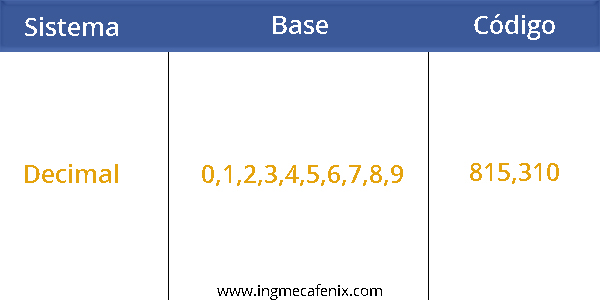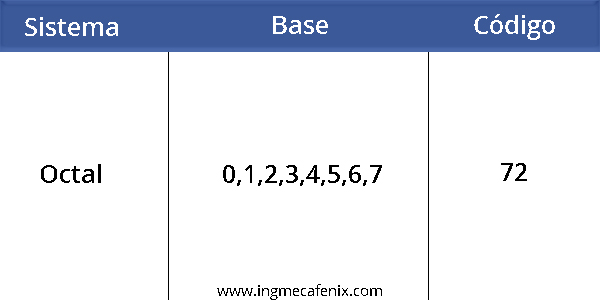# What is the difference between a system and a code?

system is a group of rules and symbols that are used to represent either data or quantities, for example we can find different types of systems, whether they are numerical such as binary, decimal, hexadecimal, octal or alphanumeric.

code is the combination of the symbols of a system, for example we can use the binary system to create a set of combinations and / or instructions for a computer to process them.## Characteristics of electronic systems and their types

One of the main characteristics of the systems is that they all have a base. This is in charge of indicating the number of different symbols it uses, for example for the binary system its base is = 2, since it only uses symbols 1 and 0.

We can find different types of systems, which we will explain below.

### Decimal system

This system is not used for electronics, but we also include it as it is considered as the main system, since it is the most used by man since ancient times. Its popularity is due to the fact that the base of this system is 10 (0,1,2,3,4,5,6,7,8,9) and they are just the fingers of both hands with which we can count on a easier.### Binary system

Practically this system is one of the simplest since its base is equal to 2 (0 and 1), it is also one of the most used because all the technology of today is built with integrated circuits which only comprise two states, 0 and 1 or high and low. Within this system we can find different types of codes such as BCD, Gray, XS3, among others.### Octal system

Its base is defined in 8 (0,1,2,3,4,5,6,7), although it has 8 symbols, which is equivalent to 1 byte, it is not used as the hexadecimal system is preferred, for 2 symbols in hexadecimal are equivalent to 1 byte, which simplifies the code further. The only advantage that this system has over the hexadecimal is that it uses the 8 equal symbols (numbers). Example the number 72 in octal is equal to 58 in decimal.v